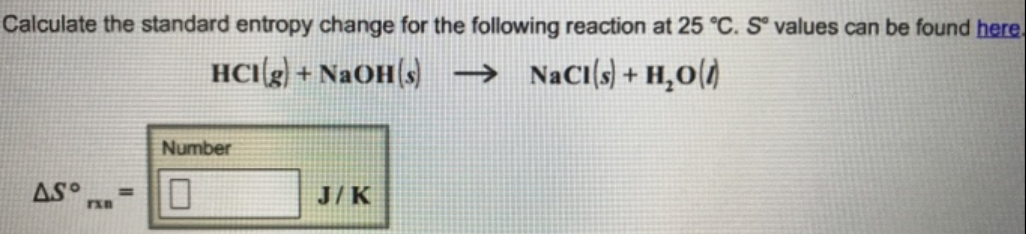# Problem: Calculate the standard entropy change for the following reaction at 25 °C. S° values can be found here. HCl (g) + NaOH (s) → NaCl (s) + H2O (l)

###### FREE Expert Solution
83% (147 ratings)###### Problem Details

Calculate the standard entropy change for the following reaction at 25 °C. S° values can be found here.

HCl (g) + NaOH (s) → NaCl (s) + H2O (l)